# 15 multiple choice calc 1

1.

Which of the following is the general solution of the differential equation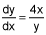? (4 points)

 y2 = x2 + C y2 = 2x2 + C y2 = 4x2 + C x2 – y2 = C

2.

The slope of the tangent line to a curve at any point (x, y) on the curve is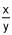. What is the equation of the curve if (4, 1) is a point on the curve? (4 points)

 x2 – y2 = 15 x2 + y2 = 15 x + y = 15 xy = 15

3.

The particular solution of the differential equation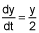for which y(0) = 80 is (4 points)

 y = 80e2t y = 80 e0.5t y = 79 + et y = 80e-0.5t

4.

The temperature of a roast varies according to Newton’s Law of Cooling: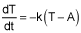, where T is the roast temperature, A is the room temperature, and k is a positive constant.

If a room temperature roast cools from 68Â°F to 25Â°F in 5 hours at freezer temperature of 20Â°F, how long (to the nearest hour) will it take the roast to cool to 21Â°F? (4 points)

 1 9 12 24

5.

Find the specific solution of the differential equation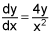with condition y(-4) = e. (4 points)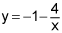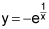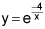None of these

1.

Choose the appropriate table for the differential equation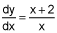. (4 points)

2.

A slope field produces (4 points)

 the graph of the differential equation the graph of the general solution to the differential equation the graph of the tangents to the differential equation the graph of the derivatives of the differential equation

3.

The differential equation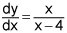. (4 points)

I. will have a slope field with negative slopes in quadrant I
II. will have a slope field with positive slopes in all quadrants
III. will produce a slope field with columns of parallel tangents

 I only II only III only None of these

4.

Which of the following differential equations is consistent with the following slope field?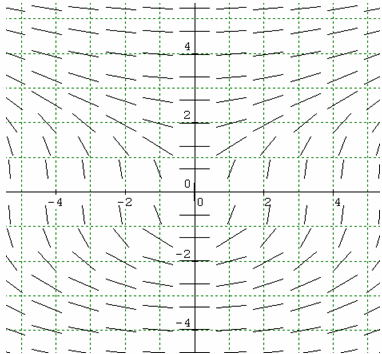(4 points)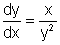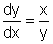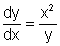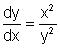5.

The differential equation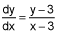. (4 points)

I. produces a slope field with horizontal tangents at y = 3
II. produces a slope field with vertical tangents at y = 3
III. produces a slope field with rows of parallel segments

 I only II only I and II III only

1.

Which of the following values would be obtained using 10 inscribed rectangles of equal width (a lower sum) to estimate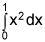? (4 points)

 0.285 0.385 1.38 2.31

2.

Which definite integral approximation formula is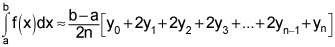? (4 points)

 Circumscribed rectangles Inscribed rectangles Mid-point rule Trapezoidal rule

3.

The estimated value of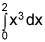, using the trapezoidal rule with 4 trapezoids is (4 points)

 3.25 5.25 4 4.25

4.

Given the table below for selected values of f(x), use 6 right rectangles to estimate the value of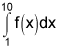. (4 points)

x 1 3 4 6 7 9 10
f(x) 4 8 6 10 10 12 16

5.

Function f(x) is positive, increasing and concave down on the closed interval [a, b]. The interval [a, b] is partitioned into 4 equal intervals and these are used to compute the left sum, right sum, and trapezoidal rule approximations for the value of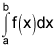Which one of the following statements is true? (4 points)

 Trapezoidal rule value < Left sum < Right sum Left sum < Trapezoidal rule value < Right sum Right sum < Trapezoidal rule value < Left sum Cannot be determined without the x-values for the partitions